## Cornu spiral and other fractal spirals

2023 February 10

Recently I have been examining discretizations of the Cornu spiral (or Euler spiral or clothoid), which have a remarkable self-similar fractal property. While this analysis of the discrete Cornu spiral is still in progress, it felt incomplete without first considering that the continuous Cornu spiral itself is fractal.

Unlike the better known logarithmic spiral1which I know as the geometric spiral but apparently this is not a standard name. The logarithmic spiral is often the most natural spiral. or Archimedean spiral2or arithmetic spiral, the Cornu spiral is described by starting a finite distance out of the spiral and then spiraling inwards. The defining property is the curvature varies linearly with the arc length from the starting point; going in either direction, then, yields a double-ended spiral. Thus the coordinates of the spiral are given by the Fresnel integral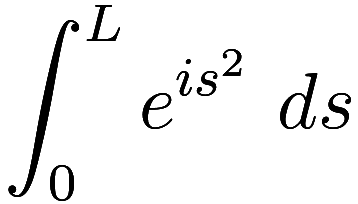which has no closed form solution. Integrating to infinity gives a finite value, the center point of the spiral. In contrast to the logarithmic or Archimedean spirals which only have finite arc length in a bounded region, and so are necessarily one-dimensional, the cornu spiral has infinite arc length and could be potentially any fractal dimension from 1 to 2.

## Cornu spiral

Let us thus compute the Minkowski or box-counting dimension of the cornu spiral. To do this, for a length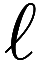we count how many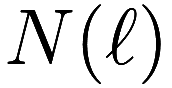squares of side-lengthit takes to cover the whole cornu spiral. For a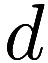-dimensional figure we expect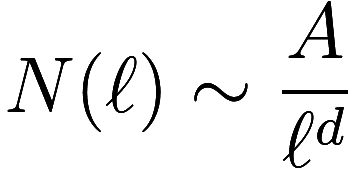(where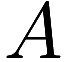is a-dimensional “area”) and thus define the Minkowski dimension as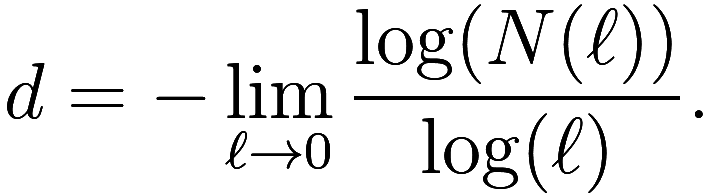In the normalized cornu spiral, the radius of curvature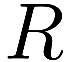at an arc length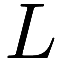is given by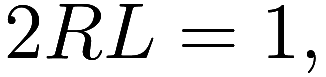asis the inverse of the curvature and curvature goes linearly with length. Whenis very large the spiral approximates a circle of radius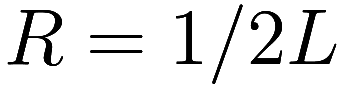around the center point. After an additional rotation around the center, our new arc length is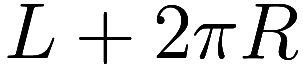, and so the new radius of curvature is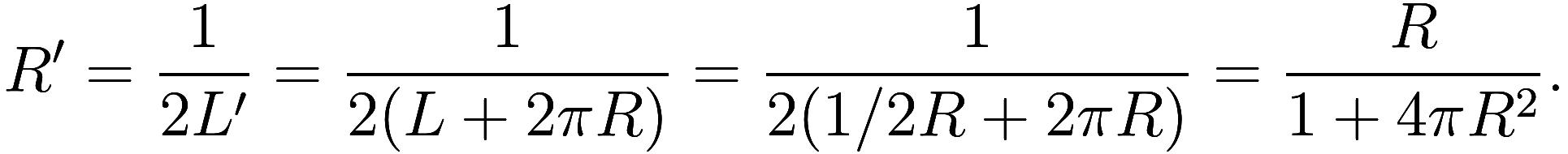So, the gap between one spiral turn and the next is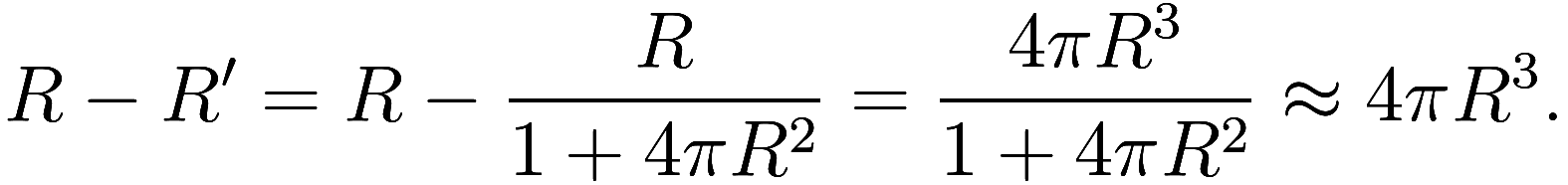This alternatively could be found using derivatives: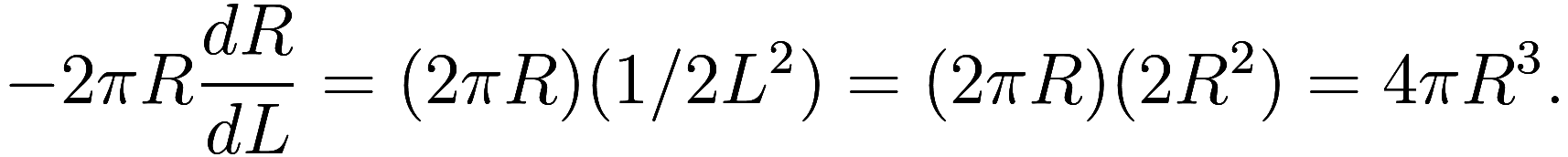Now we know enough about the shape of the spiral to count how many boxes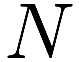are needed to cover it. Say the boxes are squares with side length. Then within some critical radius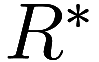boxes from consecutive turns start to overlap, and it is best to just cover the whole circle with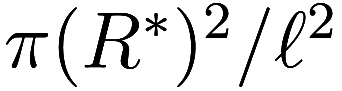boxes. Note that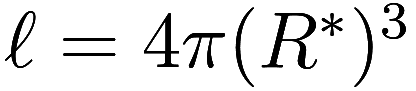, the gap between one spiral turn and the next at the critical radius.

Outside of this critical radius the number of boxes is simply the length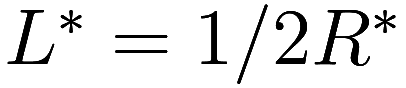of the curve divided by the widthof each box. Thus the total number of boxes is:3This is an approximation – after all, it is not an integer – but the error term goes to zero as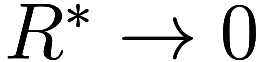, and even if the error term did not go to zero, a constant factor on the number of boxes has no effect on the fractal dimension.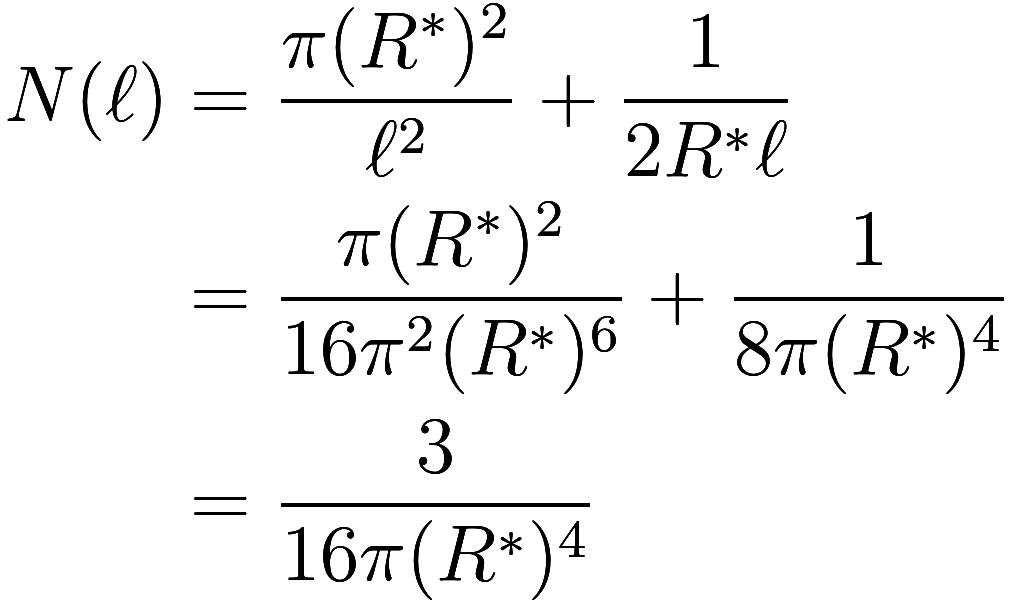Curiously we find that one-third of the boxes needed to cover the spiral are within the radius, and two-thirds are outside of that radius.

Therefore the Minkowksi fractal dimension is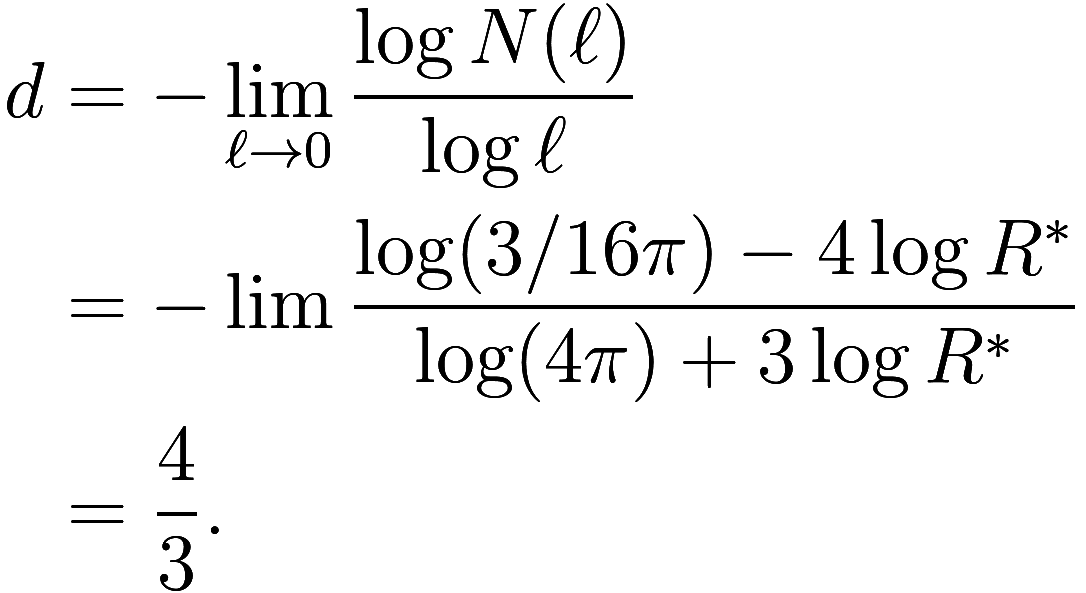## Power-law spirals

We can generalize this result, and consider similar “power law” spirals. Suppose that we have a spiral such that, at a radius offrom the center, the gap between consecutive turns scales like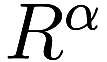; for the cornu spiral we had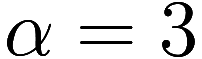. The logarithmic spiral satisfies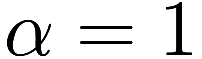, and the Archimedean spiral has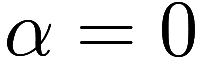. The total length of the spiral fromto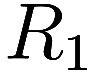is approximately4We are supposing that the spiral is always tangent to a circle centered at the spiral center, which is not correct.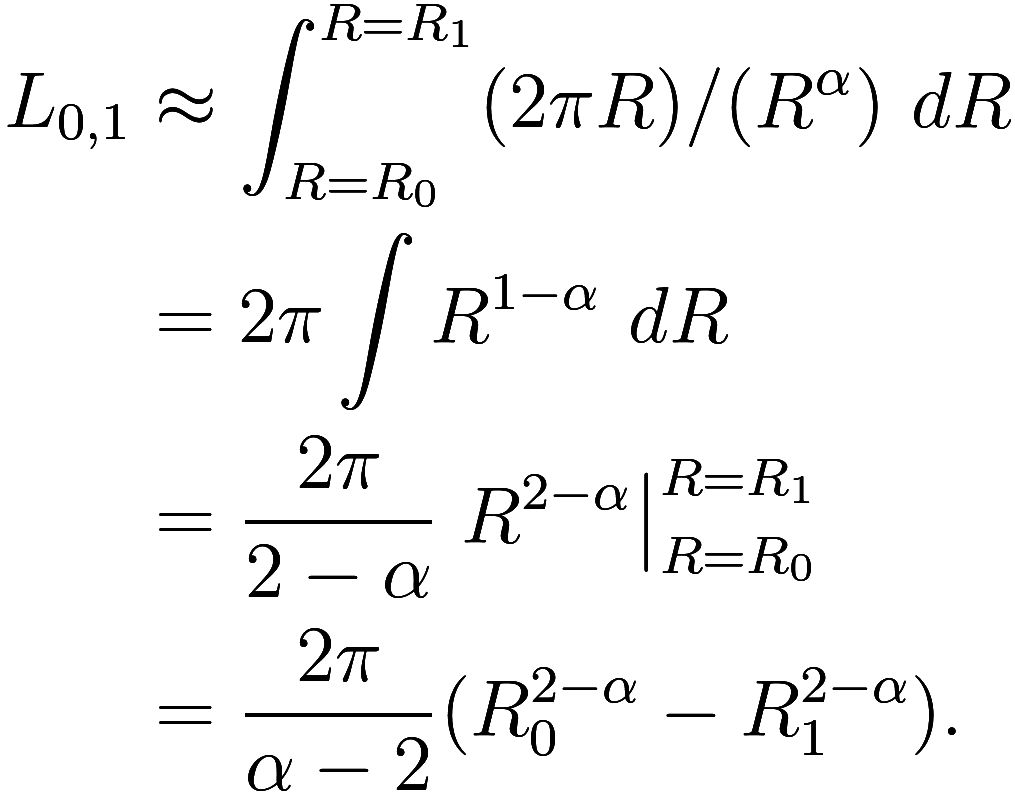where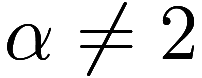. Whoops – this approximation is not always great: for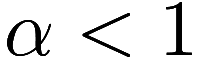, it is problematic when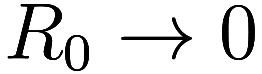, and for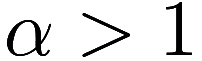when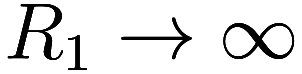, as in those cases the spiral becomes quite steep. Atit is simply off by a constant term that does not depend on. So, being more careful we find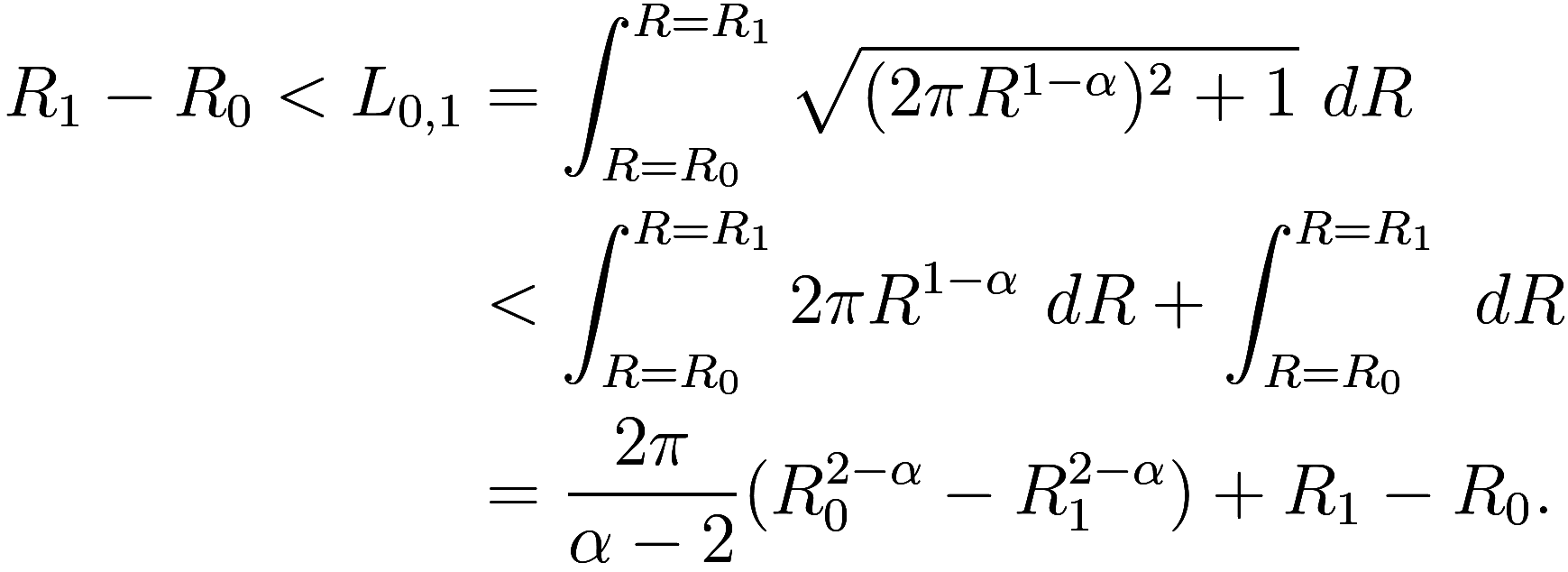where again. For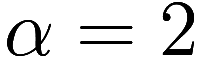we have instead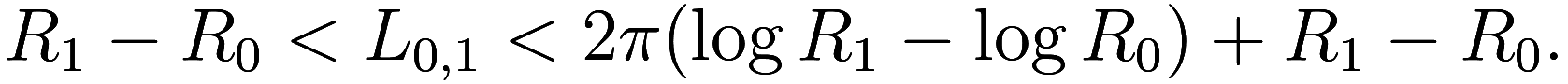We see that for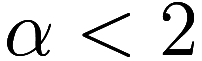the spiral has finite length as, and so it is not a fractal and has dimension 1. Only for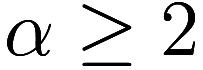do we have a fractal.

Now we proceed as before, covering the spiral with boxes of sizesuch that within a critical radiusthe whole circle is covered with no gaps, and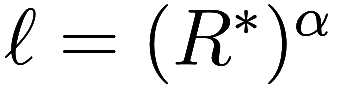. We fix some outer radius. Then the number of boxes is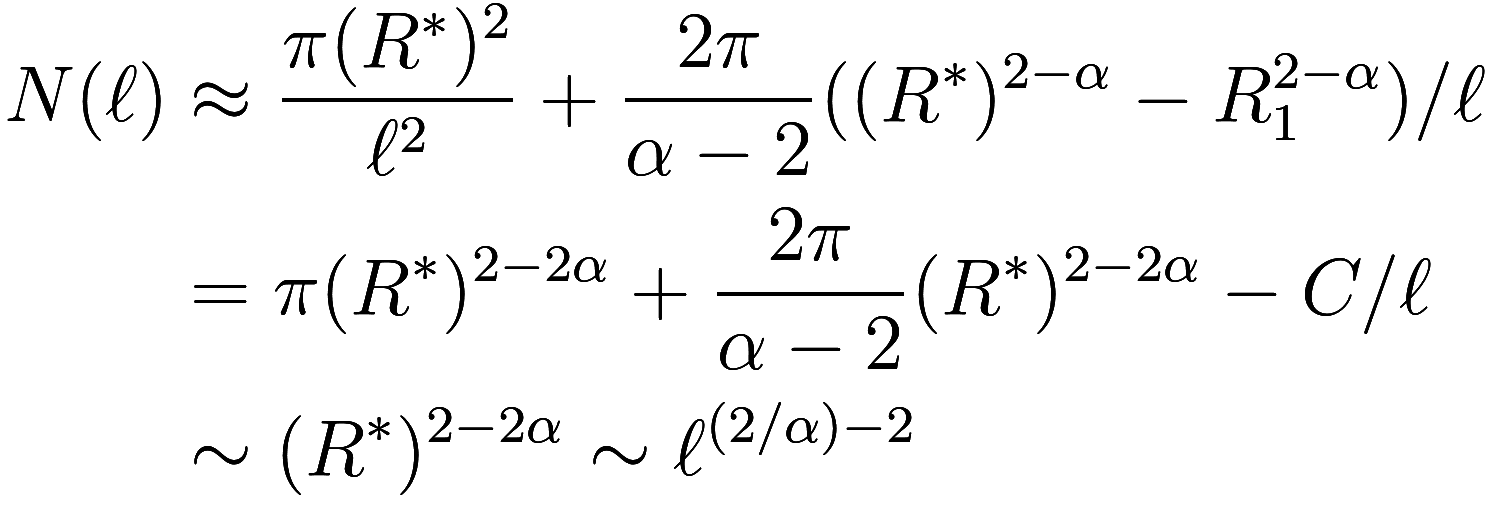Therefore the Minkowksi fractal dimension is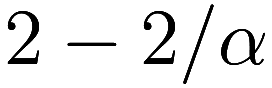. Here we neglected the error term in the integral for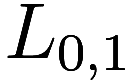as it is small forin the limit. If, we instead find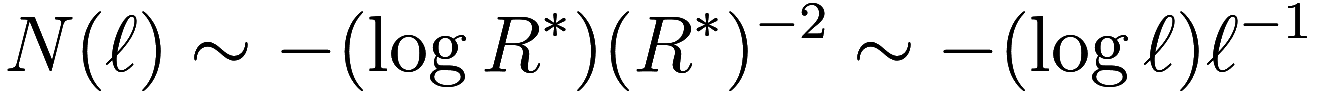so while the length of the spiral is infinite the fractal dimension is still only 1!

1. which I know as the geometric spiral but apparently this is not a standard name. The logarithmic spiral is often the most natural spiral.↩︎

2. or arithmetic spiral↩︎

3. This is an approximation – after all, it is not an integer – but the error term goes to zero as, and even if the error term did not go to zero, a constant factor on the number of boxes has no effect on the fractal dimension.↩︎

4. We are supposing that the spiral is always tangent to a circle centered at the spiral center, which is not correct.↩︎